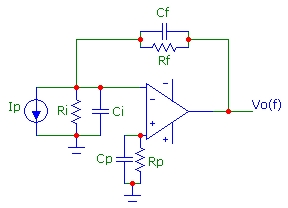# Transimpedance Noise Utility

May 11, 2012Cnoise.exe is a compiled C#.net console utility for Windows and requires .NET 4 runtime environment. Cnoise calculates the cumulative output voltage noise (up to some user-specified frequency) and the total output voltage noise (integrated out to infinite frequency) for the circuit shown. The program uses the exact transimpedance and noise-gain transfer functions and exact integrals. Cnoise.exe also displays several useful parameters for the circuit including exact 3db bandwidths and pole/zero frequencies. Although the circuit shown is a transimpedance configuration with a current source Ip, the output noise calculation applies to ANY circuit configuration with the circuit elements shown: Ri, Ci, Rf, Cf, Rp, Cp.Download cnoise.exe (21,608 bytes) (.NET 4, Digitally Signed)

Cnoise.exe can be invoked in two different ways using either 10 or 12 arguments:
```
cnoise.exe   GBW     en     in      Rf      Cf      Ri      Ci      Rp       Cp        F
or
cnoise.exe   GBW     en     in      Rf      Cf      Ri      Ci      Rp       Cp      min_pow     max_pow     stepF
```
where the circuit elements are as shown in the schematic diagram. GBW is the op-amp gain-bandwidth product (assuming a single-pole model). en is the op-amp input spectral voltage noise density and in is the op-amp input spectral current noise density. The first command-line specifies a single frequency F for the cumulative output noise calculation. For example to calculate the cumulative noise to 3 MHz:
```   cnoise.exe  19e6     6e-9    2e-15    50e3    2.6e-12    1e9     20e-12     50e3    200e-12     3e6
```
The second command line specifies a range of frequencies for cumulative noise calculation. For example to calculate cumulative noise for frequencies from 1 kHz to 10 MHz:
```   cnoise.exe  19e6     6e-9    2e-15    50e3    2.6e-12    1e9     20e-12     50e3    200e-12     3     7     1
```

The command-line output generated by the first example above is shown below. The definition of the output noise voltage components in μV are:
• Ven: voltage noise of en
• Vin: voltage noise of in flowing through Rf and Ri
• Vth: voltage noise of Rf and Ri (thermal noise sources)
• VinRp: voltage noise of in flowing through Rp
• VthRp: voltage noise of Rp (thermal noise source)
• Vtot: total RMS-summed output voltage noise
A set of plots using the parameters above created by simply importing the command-line output into Microsoft Excel is shown below.

```
C:\testfolder>cnoise  19e6   6e-9   2e-15   50e3   2.6e-12   1e9    20e-12    50e3  200e-12    3e6

GBW 19       MHz
en  6E-09    V/Sqrt(Hz)
in  2E-15    A/Sqrt(Hz)
Rf  50       kohm
Cf  2.6      pF
Ri  1000     Mohm
Ci  20       pF
Rp  50       kohm
Cp  200      pF

F0         1.636 MHz
Fz       140.852 kHz
Fp         1.224 MHz
Frp       15.915 kHz
f3db       1.627 MHz
f3dbNI    26.868 MHz
Q      0.7031

- Cumulative Output Noise (uV) to frequency 3.00E+006
Ven:      67.720
Vin:       0.131
Vth:      37.114
VinRp:     0.022
VthRp:     6.099
Vtot:     77.464

- Total Output Noise (uV) for All Frequencies
Ven:      94.015
Vin:       0.134
Vth:      38.018
VinRp:     0.022
VthRp:     6.170
Vtot:    101.599
```